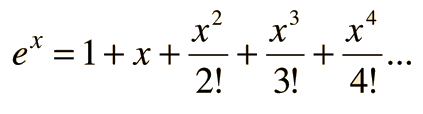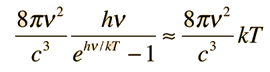# Applications of Series

Most functions can be expanded into infinite series form. This is an advantage in physical applications where one is dealing with very small numbers or a small difference between two functions. In those cases, the first few terms of a series may provide a satisfactory description of a physical phenomenon in a much simpler form than the entire function.

 Exponential Series Trigonometric Functions Binomial Expansion Taylor Series
 Natural Log Series Fourier Series
Index

 HyperPhysics****HyperMath*****Calculus R Nave
Go Back

# Exponential Series

The exponential can be expanded as an infinite series:Some applications of exponential series are:

 Planck radiation formula at low temperatures:Einstein specific heat
 Spin polarization in NMR
 Decay probability
Index

 HyperPhysics****HyperMath*****Calculus R Nave
Go Back